# Are Laplace and Poissons equations linear

Static fields are obtained as a special case of the Maxwell equations, based on a special solution of the continuity equation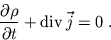The solution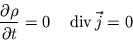describes stationary sources of the electromagnetic field, namely stationary space charges as sources of electrostatic fields and stationary currents as sources of magnetostatic fields.

5.1 Electrostatic fields
The field equations of electrostatics are for isotropic, linear media (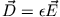)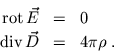They can be related to a potential equation, the Poisson equation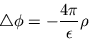summarize the given below Dirichlet or Neumann boundary conditions is to be solved.

Depending on the task, there are different solution patterns for determining electrostatic fields:

1. The field of a system of point charges:
Because of the superposition principle, we can write the field as a superposition of Coulomb fields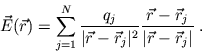2. No space charges in the volume V under consideration:
The field results exclusively from the boundary conditions, i.e. from the specification of potential (Dirichlet) or field (Neumann) on the closed interface that encloses the finite solution volume. In this volume you solve the Laplace equation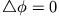in coordinates that are adapted to the geometry of the boundary condition.

Solution steps:

• Separation approach
• Fundamental system of the resulting ordinary differential equations (i.a. Special functions)
• Fundamental system of the Laplace equation (general solution)
• Determination of the coefficients by inserting the boundary conditions.

3. Space charges present:
• No finite interfaces in the volume:
The general solution to the Poisson equation is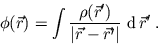Calculation of the integral in Multipole expansion, i.e. development of the Green function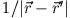in the symmetry of the charge distribution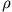adjusted coordinates.

• Finite interfaces in volume:
The general solution of the Poisson equation can be obtained with the help of Green features according to Dirichlet's or Neumann's boundary conditions.

(i) Dirichlet's boundary condition: specification of the potential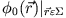on the edge surface: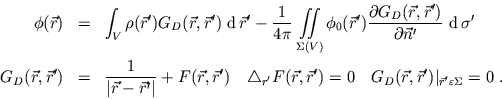Typical boundary condition for conductive surfaces: in this case the potential on the surface is constant and can be drawn in front of the integral!

(ii) Neumann's boundary condition: specification of the normal component of the field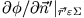on the edge surface. According to Gauss law, this is equivalent to specifying the corresponding surface charge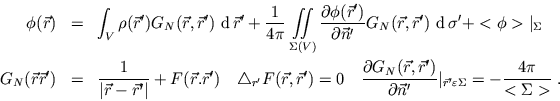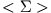: Size of the edge area,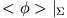: potential averaged over the edge surface.

5.2 Magnetostatic fields
The field equations of magnetostatics are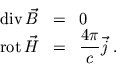One can find it with the help of the material equation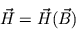solve in different ways.
1. Dia- and paramagnetics:
For linear, isotropic media are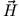- and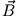Fields proportional to each other, i.e.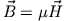. One distinguishes Diamagnetics (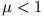) of Paramagnetics (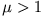).

• Vector potential for general problems:
With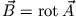is obtained in Coulombeing (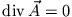)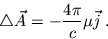The vector Poisson equation is solved component by component analogous to corresponding problems in electrostatics.

• Magnetic scalar potential for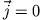:
There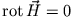, there is a magnetic scalar potential such that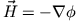. With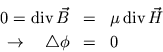one obtains a Laplace equation for the magnetic scalar potential.

2. Hard ferromagnetics:
At a hard ferromagnetic one assumes that the magnetizationis independent of the external magnetic field.

• Vector potential for general problems:
In Coulombeichung one obtains for the vector Poisson equation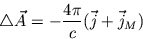with the magnetizing current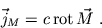The general solution to the Poisson equation is in this case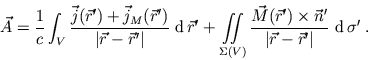The fields are obtained from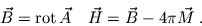• Magnetic scalar potential for:
In the absence of true currents, the Poisson equation is for the magnetic scalar potential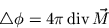to solve. The general solution is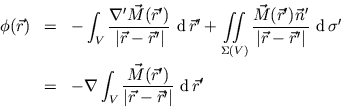The fields are obtained fromH.J. Ludde